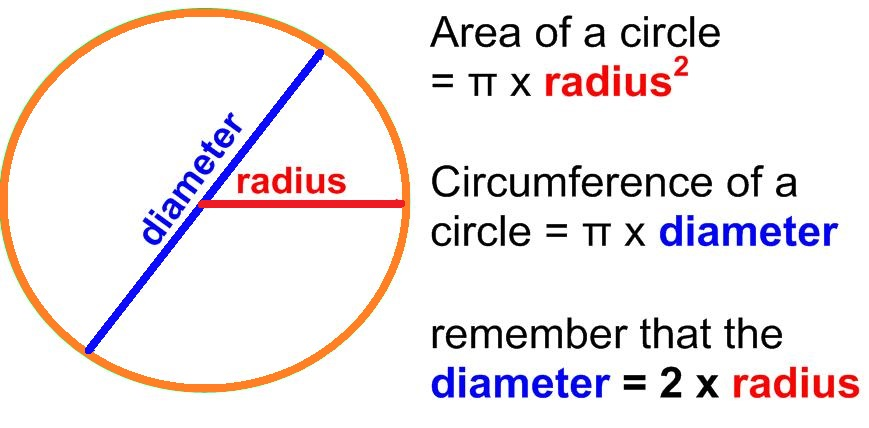# How to Calculate Circumference of Circle

## Here we will be looking at how to calculate the perimeter or the circumference of the circle.

A circle is a simple closed shape. The mathematical definition of circle is �it is a set of all points in a plane which are at a constant given distance (radius) from a given point (center)�. Explained using conic sections, �a circle is a conic section obtained by the intersection of a cone with a plane perpendicular to the cone�s symmetry axis�. Circle is a two- dimensional figure having no edge and no vertex. So as any regular two- dimensional closed figure, it has two main properties i.e. it has a perimeter and an area.

Here we will be looking at how to calculate the perimeter or the circumference of the circle. Let�s first look through the basic terminology.

• Perimeter or circumference is the continuous line forming the boundary of closed geometrical figure
• Area is the quantity that expresses the extent of a two- dimensional figure or shape in the plane.
• The center of circle is a point which is equidistant from the points on the edge of the circle.
• The radius of a circle is the distance from the center point to the point on the circle. Since, circle has radial symmetry, the distance is same of a circle.
• The straight line passing from one side to the other through the center of the circle is called the diameter.
• The number pi or ? is a mathematical constant used to calculate the circumference and the area of the circle. Being an irrational number, ? cannot be expressed as an exact numerical form but it is usually generalized as 3.14 or 22/7 for calculation purposes.

## Before the formula were created, there were other ways to calculate the circumference of the circle.

For instance, a string was used to go along the continuous boundary of the circle and it was straighten to check the perimeter it covers.

## Here, we will talk about three formulas of calculating the circumference of a circle### When the radius is given-

The formula used when the radius of a circle is given is:

• C=2?r

Here, C is the circumference and r is the radius given.

Let�s learn this using an example-

• Calculate the circumference of circle with radius 14cm

Solution – here, the radius given is, r = 14 cm

• C=2?r
• C= 2?14
• C= 2(22/7)(14) or 2(3.14)(14)
• C= 88 cm

Hence, the circumference of the circle is 88 cm.

## When the diameter is given-

The formula used when the diameter of a circle is given is-

• C=?d

Here, C is the circumference of the circle and d is the given diameter of the circle.

Let�s learn this using simple problem-

• Calculate the circumference of the circle of diameter 14 cm.

Solution- here, diameter given is, d = 14 cm

• C=?d
• C=?14
• C= 22/7(14) or 3.14(14)
• C= 44 cm

Hence, the circumference of the circle is 44 cm.

## When the area of the circle is given-

This formula might look a bit complicated, but it isn�t.�The formula used when the area of the circle is given is-

• C= 2 ? ? A

Here, C is the circumference of the circle and A is the given area of the circle.

Let�s learn this using simple problem-

• Calculate the circumference of the circle of area 14 cm

Solution- here, area given is, A = 14 cm

• C= 2 ? ? A
• C= 2 ? ? (14)
• C= 2 ? (22/7)(14) or C= 2 ? (3.14)(14)
• C= 13.26 cm

Hence, the circumference of the circle is 13.26 cm

### Related Articles

This site uses Akismet to reduce spam. Learn how your comment data is processed.

Close